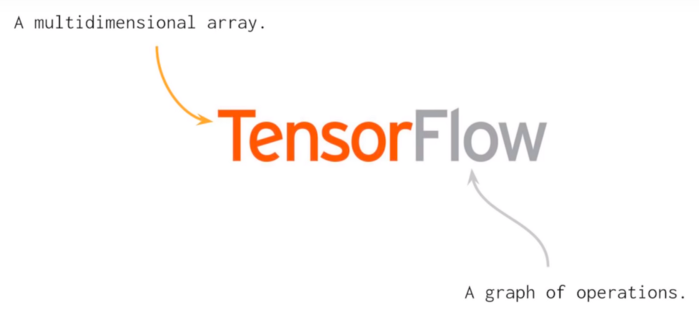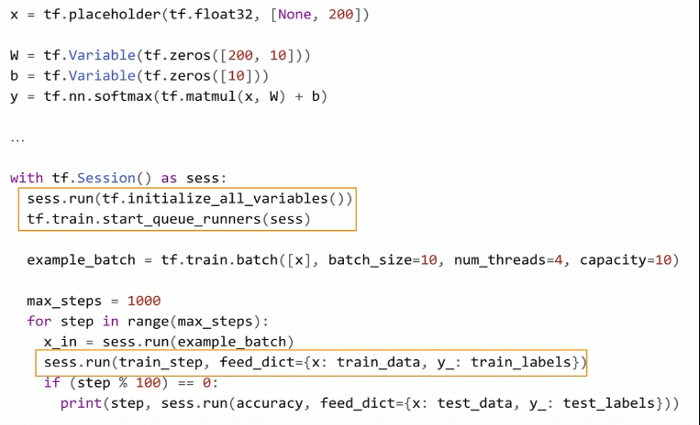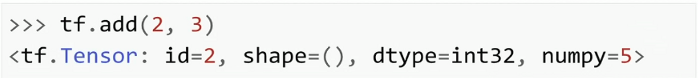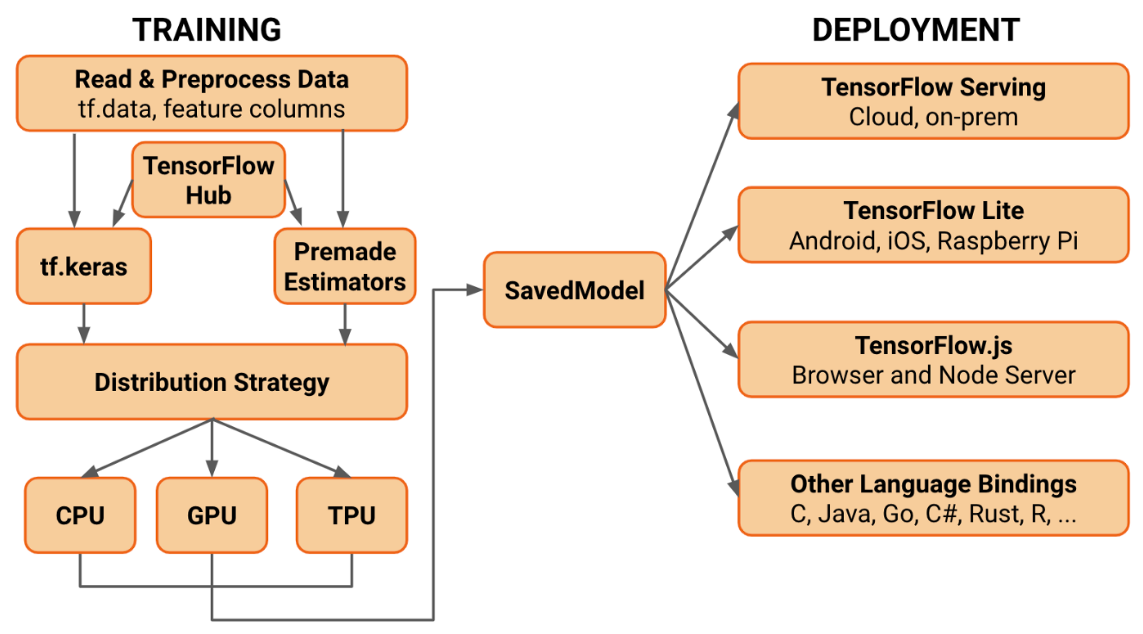InfoQ 编辑部出品——2021年度技术盘点与展望 了解详情

关于 TensorFlow 2.0 你需要了解的一切

• 2019 年 7 月 15 日
• 本文字数：6741 字

阅读完需：约 22 分钟2019 年 6 月 26 日，在 Sao Paulo 举行的PAPIs.io LATAM会议上，作为Daitan的代表，本文作者Thalles Silva举办了一个关于 TensorFlow（TF）2.0 的研讨会，并在会上探讨了一些关于 TF 2.0 的话题。研讨会的初衷是重点展示 2.0 版本同以往 1.x 版本的不同。本文回顾了会上讨论的主要内容：Keras-APIs、SavedModels、TensorBoard、Keras-Tuner 等。同时，你也可以通过 Colab notebook 来查看练习代码。

TensorFlow 2.0 简介

TensorFlow 是谷歌在 2015 年开源的一个通用高性能计算库。从一开始，TensorFlow 的主要目的就是为构建神经网络（NN）提供高性能 API。然而，借助于机器学习（ML）社区对它的兴趣以及时间上的优势，这个类库演变成了一个完整的 ML 生态系统。即时执行（Eager Execution）变为默认模式TensorFlow 1.x 代码示例TensorFlow 2.0 代码示例

TensorFlow 2.0 的代码看起来非常像 NumPy 代码。实际上，TensorFlow 和 NumPy 的对象也可以很容易地相互置换。因此，你也不用为 placeholders、Sessions 以及 feed_dictionaties 等伤脑筋了。

API 清理

TensorFlow 2.0 中，tf.keras 是推荐使用的高级 API

初级 API

# Configures the model for training.# Define the model optimizer, the loss function and the accuracy metricsmodel.compile(optimizer='adam', loss='sparse_categorical_crossentropy', metrics=['accuracy'])model.summary()

Keras-Tuner

Keras-Tuner是一个专门为 Keras 模型优化超参数的独立库。在本文写作的时候，该类库仍处于 pre-alpha 阶段，即便如此，它已经可以在 Colab 上很好地同 tf.keras 以及 TensorFlow2.0 beta 一起工作。

def build_model(hp):    # define the hyper parameter ranges for the learning rate, dropout and hidden unit    hp_units = hp.Range('units', min_value=32, max_value=128, step=32)    hp_lr = hp.Choice('learning_rate', values=[1e-2, 1e-3, 1e-4])    hp_dropout = hp.Choice('dropout', values=[0.1,0.2,0.3])
# compile and return the model model.compile(optimizer=keras.optimizers.Adam(hp_lr), loss='sparse_categorical_crossentropy', metrics=['accuracy']) return model # create a Random Search tuner tuner = RandomSearch( build_model, objective='val_accuracy', # define the metric to be optimized over max_trials=3, executions_per_trial=1, directory='my_logs') # define the output log/checkpoints folder # start hyper-parameter optmization search tuner.search(x_train, y_train, epochs=2, validation_data=(x_test, y_test))

高级 API

class Model(tf.keras.Model):  def __init__(self):    # Define the layers here    super(Model, self).__init__()    self.conv1 = Conv2D(filters=8, kernel_size=4, padding="same", strides=1, input_shape=(IMAGE_HEIGHT,IMAGE_WIDTH,IMAGE_DEPTH))    self.conv2 = Conv2D(filters=16, kernel_size=4, padding="same", strides=1)    self.pool = MaxPool2D(pool_size=2, strides=2, padding="same")    self.flat = Flatten()    self.probs = Dense(units=N_CLASSES, activation='softmax', name="output")    def call(self, x):    # Define the forward pass    net = self.conv1(x)    net = self.pool(net)    net = self.conv2(net)    net = self.pool(net)    net = self.flat(net)    net = self.probs(net)    return net    def compute_output_shape(self, input_shape):    # You need to override this function if you want to use the subclassed model    # as part of a functional-style model.    # Otherwise, this method is optional.    shape = tf.TensorShape(input_shape).as_list()    shape[-1] = self.num_classes    return tf.TensorShape(shape)

• 正向传播

• 损失函数评估

• 反向传播

• 梯度下降

配置 TensorBoard

# create the tensorboard callback tensorboard = TensorBoard(log_dir='logs/{}'.format(time.time()), histogram_freq=1)
# train the model model.fit(x=x_train, y=y_train, epochs=2, validation_data=(x_test, y_test), callbacks=[tensorboard])
# launch TensorBoard %tensorboard --logdir logs

• EarlyStopping：如同字面含义，它设置了一条规则，当被检测的量化值停止改善时就结束训练。

• ReduceLROnPlateau：当评估值停止改善的时候就降低学习率。

• TerminateOnNaN：当遇到一个 NaN 损失的时候就回调结束训练。

• LambdaCallback：一个创建样本的回调，自定义回调正在开发过程中。

提升 EagerCode 的性能

@tf.function  def train_step(images, labels):
with tf.GradientTape() as tape: # forward pass predictions = model(images) # compute the loss loss = cross_entropy(tf.one_hot(labels, N_CLASSES), predictions) # get the gradients w.r.t the model's weights gradients = tape.gradient(loss, model.trainable_variables) # perform a gradient descent step optimizer.apply_gradients(zip(gradients, model.trainable_variables)) # accumulates the training loss and accuracy train_loss(loss) train_accuracy(labels, predictions)

模型保存和恢复

TensorFlow 1.x 中缺少的另外一项标准就是我们如何为产品保存和装载模型。TensorFlow 2.0 试图通过定义一个单一 API 来解决这个问题。

TF 2.0 并没有采用保存模型的很多方法，它反而标准化了一个叫做 SavedModel 的抽象。

# serialize your model to a SavedModel object  # It includes the entire graph, all variables and weights  model.save('/tmp/model', save_format='tf')

TensorFlow 生态系统整合了 SavedModels，也就是说，你可以将它部署到很多设备中，诸如手机、边缘设备以及服务器等。TF-Lite 转化

# create a TensorFlow Lite converter   converter = tf.lite.TensorFlowLiteConverter.from_keras_model(model)
# performs model quantization to reduce the size of the model and improve latency converter.optimizations = [tf.lite.Optimize.OPTIMIZE_FOR_SIZE]
tflite_model = converter.convert()

TensorFLow.js 转化

!tensorflowjs_converter \      --input_format=tf_saved_model \      --saved_model_tags=serve \      --output_format=tfjs_graph_model \      /tmp/model \      /tmp/web_modelview raw

结语

2019 年 7 月 15 日 13:549182评论 1 条评论2019 年 07 月 17 日 09:13

• 深度学习指南：在 iOS 平台上使用 TensorFlow

本文主要介绍TensorFlow背后的设计思路、如何利用其训练一套简单的分类器，以及如何将上述成果引入您的iOS应用。

• 离线评估：常用的推荐系统离线评估方法有哪些？

在推荐系统这个行业，所有人都在谈效果。那这个所谓的“效果”到底指的是什么呢？我们一般用什么方法来衡量这个“效果”呢？

2020 年 12 月 7 日

• 谷歌官方解读：TensorFlow 2.0 哪些新特性值得关注？

2018 年 11 月，TensorFlow 在庆祝 3 岁生日时回顾了其多年来增加的功能。 对于即将发布的 TensorFlow 2.0，TensorFlow 团队感到非常兴奋。相比于旧版本，这个被团队视为另一重要里程碑的版本又新增了哪些功能呢？

• 如何基于 TensorFlow 2.0 用十行代码实现性能最佳的 NLP 模型？

一文手把手教你如何基于 TensorFlow 2.0，仅用十行代码即可实现性能最佳的自然语言处理模型。

• 介绍 Gluon 这一易于使用的灵活深度学习编程接口

在 Gluon 中，您可以使用简单、清晰和简洁的代码定义神经网络。

• Embedding+MLP：如何用 TensorFlow 实现经典的深度学习模型？

你知道微软在2016年提出的深度学习模型Deep Crossing吗？它就属于经典的Embedding+MLP模型。

2020 年 11 月 18 日

• 深入浅出 TensorFlow（六）TensorFlow 高层封装

为了让TensorFlow使用起来更加灵活，更加方便，可以使用一些高级封装。本文将介绍TensorFlow的四种主要封装：TensorFlow-Slim、tf.contrib.learn（之前也被称为skflow）、TFLearn、Keras；同时还将通过其中常用的三种方式在MNIST数据集上实现卷积神经网络。

• 11｜深度学习（上）：用 CNN 带你认识深度学习

要构建出能够实现图像分类的CNN，我们通常需要把“卷积+池化”的组合重复搭建几次，形成深度卷积网络。

2021 年 9 月 22 日

• 如何用几行代码运行 40 个回归模型

这篇文章教你如何使用 Lazy Predict 运行超过 40 个机器学习模型进行回归项目。

• 2019 年你不可不知的十大 Python 库

本文将讨论 Python 中的一些顶级库，开发人员可以使用这些库来解析、清理和表示数据，并在现有应用中实现机器学习。

• 如何用 Python 构建一个决策树

本文最初发布于Medium网站，经原作者授权由InfoQ中文站翻译并分享。决策树是一个经久不衰的话题。

• Inside Tensorflow 之：tf.keras part1

其实在Layer中也可以实现losses和metrics的追踪功能。

• 都在关心 TensorFlow 2.0，那我手里基于 1.x 构建的程序怎么办？

本文将跟大家分享作者在处理TensorFlow适配和版本选择问题方面的经验，希望对你有所帮助。

• 用户登录（二）：创建 sessionKey model 并完善用户登录

2020 年 8 月 20 日

• 神经网络的训练：初始化

2020 年 4 月 16 日

• TensorFlow 发布 1.12.0 版本，改善 XLA 稳定性和性能

近日，TensorFlow发布1.12.0版本，对主要功能进行了一些改进，并修复了一些错误，如Keras模型现在可以直接导出为SavedModel格式等。

• 四种常见 NLP 框架使用总结

本文主要针对于如何使用框架实现自定义模型，帮助读者快速了解框架的使用方法。

• 13｜深度学习（下）：3 招提升神经网络预测准确率

深度学习的性能优化和其它普通的机器学习模型类似，我们也可以从数据处理、网络模型本身，以及如何对神经网络进行参数调优这三个主要方面去考虑。

2021 年 9 月 27 日

ARTS 打卡计划

一点思考|工作十几年了，竟从未用过do-while！

Java